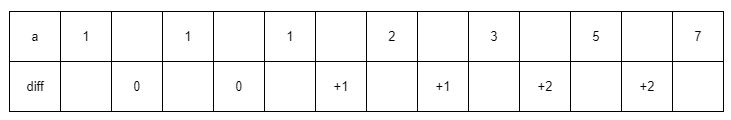## Solution:

First. We can change $a_x + a_y = a_z + a_w$ to $a_x - a_w = a_z - a_y$. So the problem becomes a problem of finding two pairs so that their differ of each pair are equal.

So how many different differ are there? In fact, there are at most 2500 differs. Consider the different differ is $(0,1,2,3,4,...)$. So that the minimum $a$ should be $(1,1,2,4,7,11,...)$.

We can find that we already exceed the maximum value of $a$ when the differ is 2500.

And noted that, every number can only be used once. So when $n\geq 5000$, there must be an answer.In case to avoid two adjacent differs are the same. The n should be 2 times of the number of all possible differs.

So, in case $n\geq 5000$. For sorted $a$, one of the differ of two adjacent numbers will appear at least 3 times (So at least 4 numbers are involved).

In case $n<5000$. We find out all the differs of any two numbers by brute force.

## Code:蜀ICP备19018968号